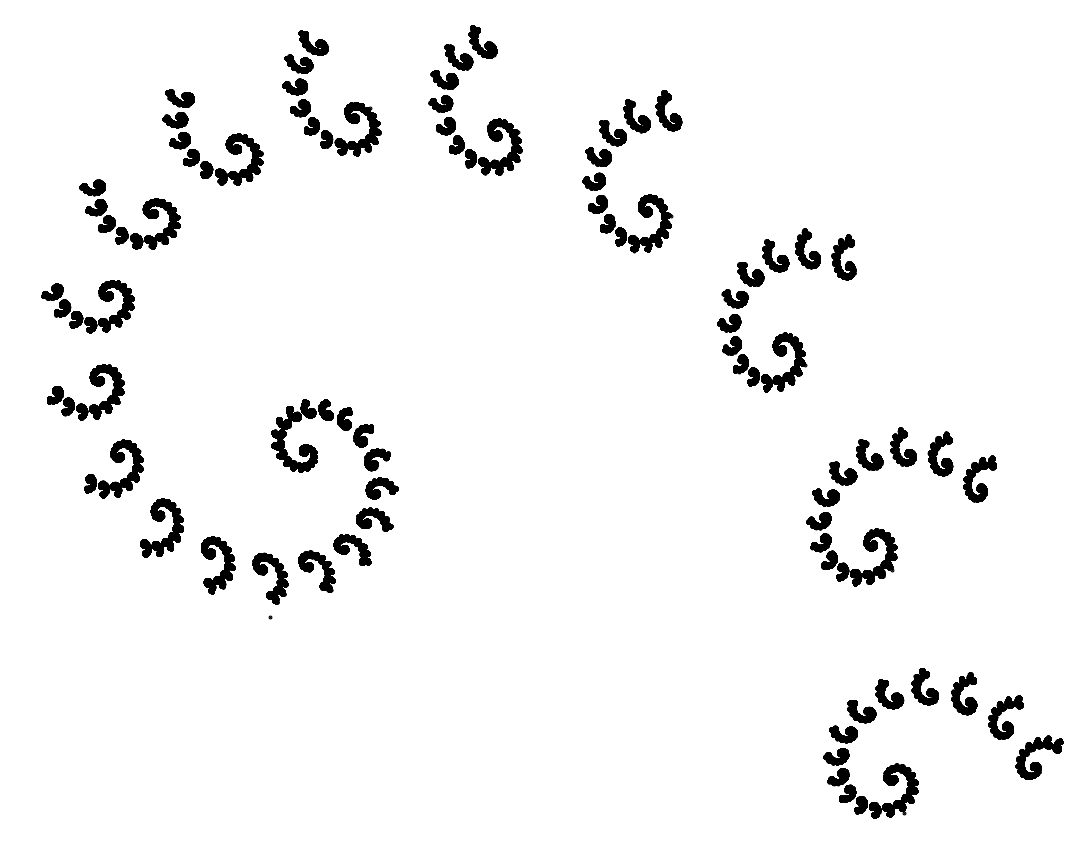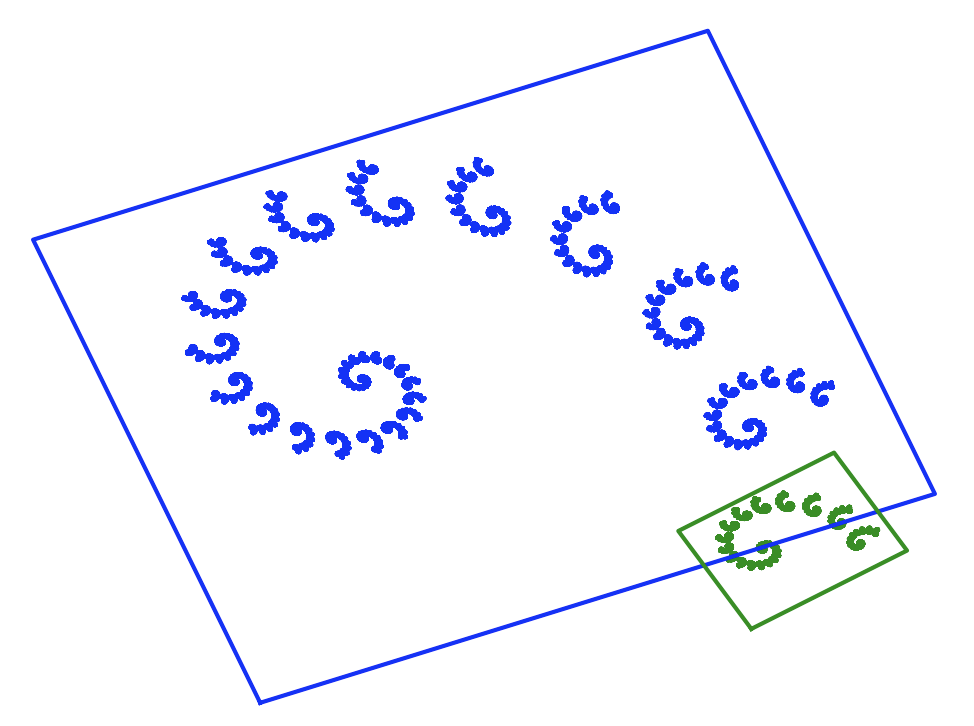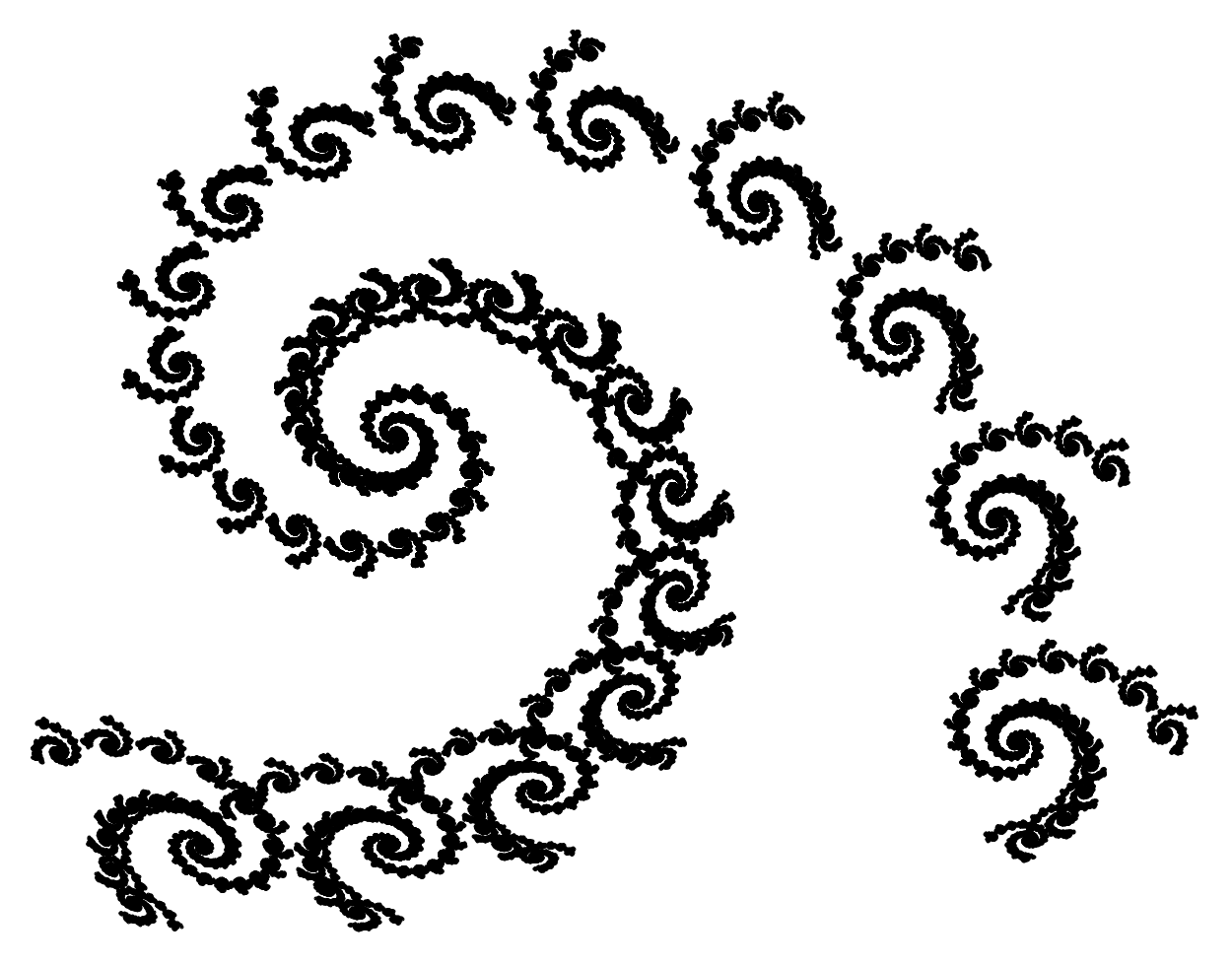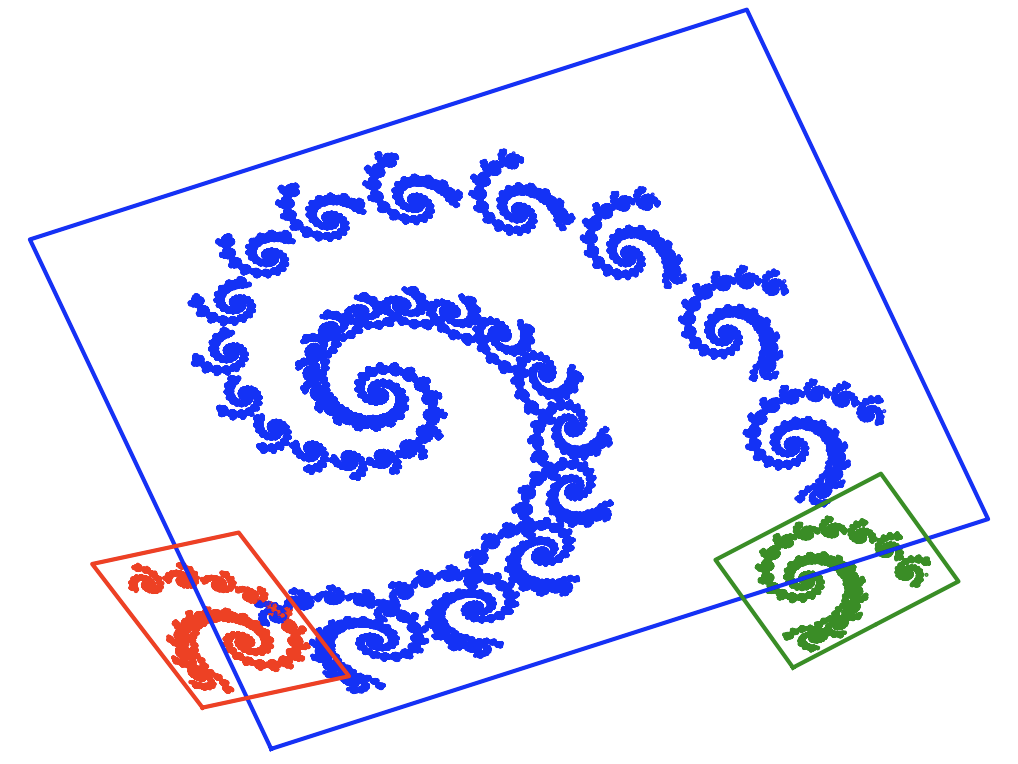## 4.5 SpiralUsing rotations, you can get very interesting spiral shapes. An interesting effect can be obtained with just two transformations:

• Transformation 1: rescale the figure by 90% and rotate by 20 degrees.
• Transformation 2: rescale the figure by 10%, rotate by 20 degrees and shift by 1 in the horizontal axis.Transformations for the spiral. Color indicates the images resulting from applying successive transformations.

Graph obtained with the chaos game algorithm with probabilities $$\pi = [0.9; 0.1]$$.

$f_1(x, y) = \begin{bmatrix} 0.787 & -0.424 & 1.758647 \\ 0.242 & 0.859 & 1.408 \end{bmatrix} [x \ y \ 1]^T$ $f_2(x, y) = \begin{bmatrix} 0.181 & -0.136 & 6.086 \\ 0.090 & 0.181 & 1.568 \end{bmatrix} [x \ y \ 1]^T$By adding additional transformations, we can enrich this spiral with a second braid.Graph obtained with the chaos game algorithm with probabilities $$\pi = [0.7; 0.15; 0.15]$$.
$f_1(x, y) = \begin{bmatrix} 0.787 & -0.424 & 1.758647 \\ 0.242 & 0.859 & 1.408 \end{bmatrix} [x \ y \ 1]^T$ $f_2(x, y) = \begin{bmatrix} -0.121 & 0.257 & -6.721 \\ 0.151 & 0.053 & 1.377 \end{bmatrix} [x \ y \ 1]^T$ $f_3(x, y) = \begin{bmatrix} 0.181 & -0.136 & 6.086 \\ 0.090 & 0.181 & 1.568 \end{bmatrix} [x \ y \ 1]^T$